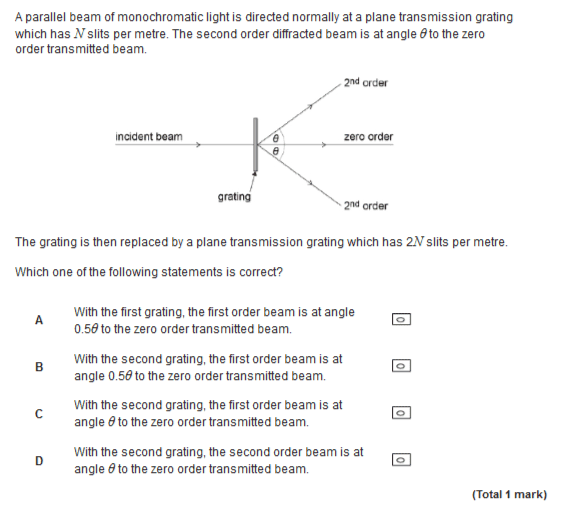# Transmission grating Nλ=dsinθ

• maxelcat

## Homework Statement

This is a question we have been doing at school. The answer is C. I think A is correct as well.## Homework Equations

I have used nλ=dsinθ and put in test values.
I guess I should be able to do this by just 'inspecting the question' but the sin confused me. So I put in some test values as below.

## The Attempt at a Solution

When I used 600nm for wavelength and 100,000 lines per meter the first order and second orders are at 3.4398 degrees 6.8921degrees. Doubling N then gives 6.8921 and 13.8865 degrees. This suggests that a is right... so what am I doing wrong please

#### Attachments

Last edited by a moderator:
I think you are confusing the number of lines ##N## with the order of a given line. Suppose you were to write the grating equation as ##d \sin \theta = m \lambda## where ##m## is the order of the line (here ##m=2##). The separation between lines, ##d##, is halved when the number of lines ##N## is doubled.

Thanks for your reply. I don't think i am confusing N and n. When N is doubled, d is halves like you say. Small n (or m if you like) is the feinge number. So ...

For fixed N then theta for n=1 seems to be half what it for n=2... ie a is right... which it isn't according to the mark scheme

But... i have sorted it now ... a is wrong because the angle doesn't necessarily double as you go from n=1 to n=2 - at small angles it approximately does, at larger angles (like in the diagram) it doesn't

So which answer do you think is correct?

C -according to the mark scheme!

(c) is indeed the correct answer. Do you see why?